# 【深入理解C++11】第四章 新手易学，老兵易用

#### 4.2 auto类型推导

auto在C++11中有了新的定义，例如auto a = 1;a的类型将会被推导为int类型，也可以用在例如，将会在4.4节中的追踪返回类型的函数声明，以及7.3节的lambda与auto的配合使用等等。

1. 不可以作为函数入参类型。
2. 不可以非静态成员变量。
3. 不可以使用auto数组
4. 不可以作为模板参数
见以下示例：
void func(auto x = 1) {} //1. 无法通过编译

struct str {
auto var = 0;	     //2. 非静态成员,无法通过编译
};

char x;
auto z = x;			//3. auto数组,无法通过编译

vector<auto> v = { 1 };	//4. auto模板参数,无法通过编译



#### 4.3 decltype

##### 4.3.1 typeid与decltype

	//C++11新增hash_code
cout << typeid(Node).hash_code() << endl; //打印一堆数字

float a;
double b;
//声明c的类型
decltype(a + b) c;
cout << typeid(c).name() << endl;		//打印:double


##### 4.3.2 decltype的应用

vector<int> vec;
typedef decltype(vec.begin()) vectype;
for (vectype i = vec.begin(); i < vec.end(); ++i) {}

template<typename T1, typename T2>
void sum(T1 &t1, T2 &t2, decltype(t1 + t2) &s)
{
s = t1 + t2;
}


namespace gxlemon
{
template<typename>
struct result_of;

template<typename F, typename ...Args>
struct result_of<F(Args...)>
{
typedef decltype(std::declval<F>()(std::declval<Args>()...)) type;
};
}

//使用如下
typedef double(*pFunc)();
//要求模板参数 是一种类型,而不是具体的某个对象
//这样a就是该函数类型的返回类型
gxlemon::result_of<pFunc()>::type a;

##### 4.3.3 decltype推导四规则
		int i;
decltype(i) a;
decltype((i)) b;  //“b”: 必须初始化引用


1. 如果e是一个没有带括号的标记符表达式，或者类成员访问表达式，那么decltype(e)就是e的实体类型。另外e如果是一个重载函数，将会编译错误。
2. 否则，假设e是类型T，如果e是一个将亡值（xvalue），那么decltype(e）为T&&。
3. 否则，假设e是类型T，如果e是一个左值，则decltype(e）为T&。
4. 否则，假设e是类型T，则decltype(e）为T。
简单举例解释一下标记符表达式int arr，arr就是一个标记符表达式，例如arr + 0, arr,都不是标记符表达式。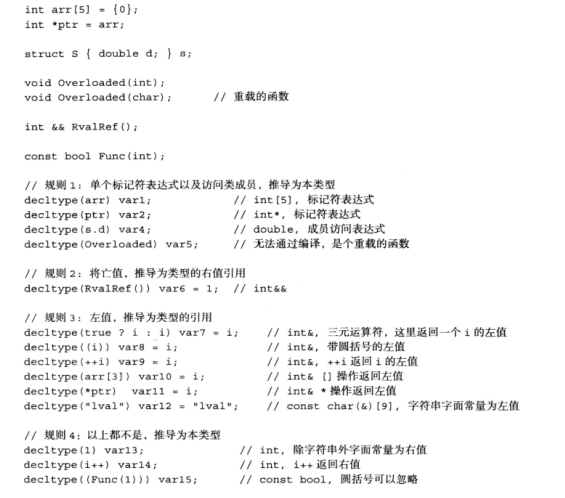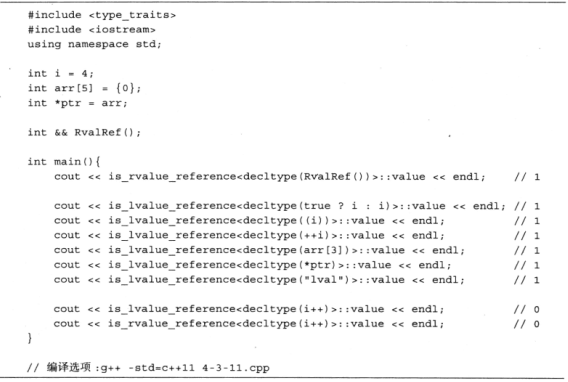##### 4.3.4 cv限制符的继承与冗余的符号

		const int ic = 0;
volatile int iv;

struct S { int i; };

const S a = { 0 };
volatile S b;
volatile S *p = &b;

cout << "is_const<decltype(ic)>::value " << is_const<decltype(ic)>::value << endl;
cout << "is_volatile<decltype(iv)>::value "<< is_volatile<decltype(iv)>::value << endl;
cout << "is_const<decltype(a)>::value " << is_const<decltype(a)>::value << endl;
cout << "is_volatile<decltype(b)>::value " << is_volatile<decltype(b)>::value << endl;
cout << "is_const<decltype(a.i)>::value " << is_const<decltype(a.i)>::value << endl;
cout << "is_volatile<decltype(b.i)>::value " << is_volatile<decltype(b.i)>::value << endl;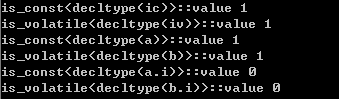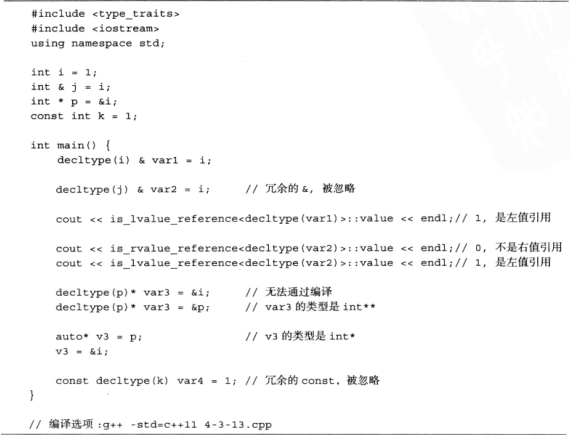#### 4.4 追踪返回类型

##### 4.4.1 追踪返回类型的引入

//C++编译器规则：变量使用前必须声明，所以可以追踪返回类型，见下例子
template<typename T1, typename T2>
decltype(t1 + t2) sum1(T1 t1, T2 t2)
{
return t1 + t2;
}

template<typename T1, typename T2>
auto  sum2(T1 t1, T2 t2) -> decltype(t1 + t2)
{
return t1 + t2;
}

##### 4.4.2 使用追踪返回类型的函数

//可能是一个面试题
int(*(*pf())())()
{
return nullptr;
}

//		   -> 返回一个a函数的指针的函数 -> 返回一个函数指针a
auto pf1() -> auto (*)() -> int(*)()
{
return nullptr;
}


//声明函数指针类型
typedef int(*PFUNC)();

//一个函数 返回int
int fun()
{
return 1;
}

//返回一个函数指针
PFUNC funRetPF()
{
return fun;
}

//声明返回一个函数指针的类型
typedef int(*(*PFUNCRETFUN)())();

//可能是一个面试题
int(*(*pf())())()
{
PFUNCRETFUN f = funRetPF;
return f;
}

//		   -> 返回一个a函数的指针的函数 -> 返回一个函数指针a
auto pf1() -> auto (*)() -> int(*)()
{
PFUNCRETFUN f = funRetPF;
return f;
}
cout << std::is_same<decltype(pf1), decltype(pf)>::value << endl;

cout << "pf1: " << pf1()()() << endl;
cout << "pf : " << pf()()() << endl;



#### 4.5 基于范围的for循环

std::vector<int> gVecs;

void fun()
{
for (int i = 0; i < 100; ++i)
{
gVecs.pop_back();
}
}

int main()
{
for (int i = 0; i < 200; ++i)
{
gVecs.push_back(i);
}
//迭代
for (int val : gVecs)
{
if (val == 50)	//触发线程删除部分元素
{
thd.detach();
}
cout << val << " ";
}
getchar();
return 0;
}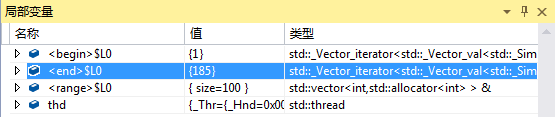07-315086

#### 最新版Redis安装配置教程（Windows+Linux）07-308075

#### YOLO系列算法精讲：从yolov1至yolov4的进阶之路（呕心沥血2万字超全整理，建议收藏！）©️2020 CSDN 皮肤主题: 大白 设计师: CSDN官方博客点击重新获取扫码支付1.余额是钱包充值的虚拟货币，按照1:1的比例进行支付金额的抵扣。
2.余额无法直接购买下载，可以购买VIP、C币套餐、付费专栏及课程。余额充值